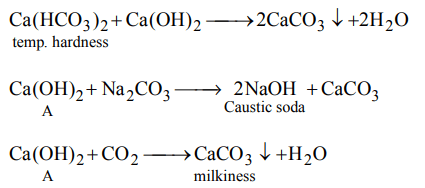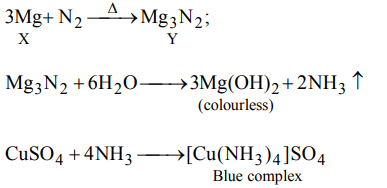## The s-Block Elements Questions and Answers Part-14

1. Which of the following peroxides is thermally most stable?
a) $SrO_{2}$
b) $MgO_{2}$
c) $CaO_{2}$
d) $BaO_{2}$

Explanation: The stability of the peroxide increases as the size of the metal increases

2. Mark the compound which does not contain calcium carbonate?
a) Fluorspar
b) Iceland spar
c) Marble
d) Limestone

Explanation: Fluorspar is CaF2 while other compounds listed are different crystalline forms of CaCO3 .

3. Thermal stability of alkaline earth metal carbonates decreases in order
a) $BaCO_{3}>SrCO_{3}>CaCO_{3}>MgCO_{3}$
b) $BaCO_{3}>SrCO_{3}>MgCO_{3}>CaCO_{3}$
c) $CaCO_{3}>SrCO_{3}>MgCO_{3}>BaCO_{3}$
d) None of these

Explanation: $BaCO_{3}>SrCO_{3}>CaCO_{3}>MgCO_{3}$

4. Which of the following metal carbonates decomposes on heating ?
a) $MgCO_{3}$
b) $Na_{2}CO_{3}$
c) $K_{2}CO_{3}$
d) $Rb_{2}CO_{3}$

Explanation: MgCO3 decomposes on heating to give MgO and CO2 while alkali metal carbonates do not.

5. Which of the following is incorrect?
a) Mg burns in air releasing dazzling light rich in UV rays
b) $CaCl_{2}.6H_{2}O$   when mixed with ice gives freezing mixture
c) Mg cannot form complexes
d) Be can form complexes due to its very small size

Explanation: Mg cannot form complexes

6. Chemical A is used for water softening to remove temporary hardness. A reacts with $Na_{2}CO_{3}$ to generate caustic soda. When $CO_{2}$ is bubbled through A, it turns cloudly. What isthe chemical formula of A
a) $CaCO_{3}$
b) CaO
c) $Ca\left(OH\right)_{2}$
d) $Ca\left(HCO_{3}\right)_{2}$

Explanation:7. Blanc fire used in paints is
a) finely divided $BaSO_{4}$
b) paste of $Mg\left(OH\right)_{2}$
c) suspension of slaked lime
d) $MgCl_{2}.5MgO.5H_{2}O$

Explanation: finely divided $BaSO_{4}$

8. Mg is an important component of which biomolecule occurring extensively in living world?
a) Haemoglobin
b) Chlorophyll
c) Florigen
d) ATP

Explanation: Chlorophyll

9. A metal X on heating in nitrogen gas gives Y. Y on treatment with $H_{2}O$  gives a colourless gas which when passed through $CuSO_{4}$  solution gives a blue colour. Y is
a) $Mg\left(NO_{3}\right)_{2}$
b) $Mg_{3}N_{2}$
c) $NH_{3}$
d) MgOa) $\left(COO\right)_{2}Ca$
b) $\left(COO\right)_{2}Ba$
c) $\left(COONa\right)_{2}$
d) $\left(COO\right)_{2}Mg$
Explanation: $\left(COO\right)_{2}Ca$